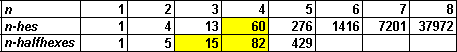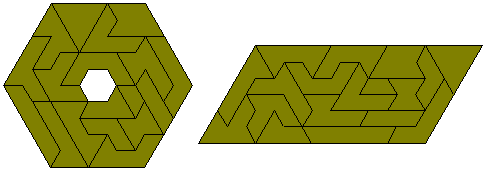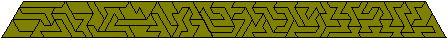An n-he is a shape formed from a polyhex by the removal of half of each hexagon in such a way that the remaining pieces are connected. Brendan Owen has suggested these and has produced the following table. An alternative definition would be to combine half hexagons together in an hexagonal grid. The lower line in the table below gives the numbers for these sets.For an alternative method of joining half hexagons see Polyflaptiles. Also see Other Hexagon Based Forms

The one monohe, the four dihes and the thirteen trihes can be combined to form a triangle.They can also form a number of other shapes as shown here.The 13 trihes can form a number of constructions. Brendan Owen has found 1062 solutions to the figure at the left.There are 22 one-sided trihes and Brendan Owen has found a number of patterns which can be made with the set. Brendan also found that the figure at the right has no solution.A number of other symmetrical figures made with the set are shown here.There 60 tetrahes as shown in this trapezium. If we divide each piece up into twelve triangle then we find that there is a odd number of pieces with a colour excess of two triangle. This restricts the type of symmetric figures which could be made.The alternative definition of polyhes gives 15 pieces with three half hexagons and 26 pieces for the one-sided set.The 82 pieces with four half hexagons are seen here in hexagon with a single hole and two parallelograms made by Roel Huisman.Roel has also found a solution the the problem of finding a nine-fold replica of one of the pieces.Isoperimetric Polyhes

There are 19 perimeter 12 polyhes.